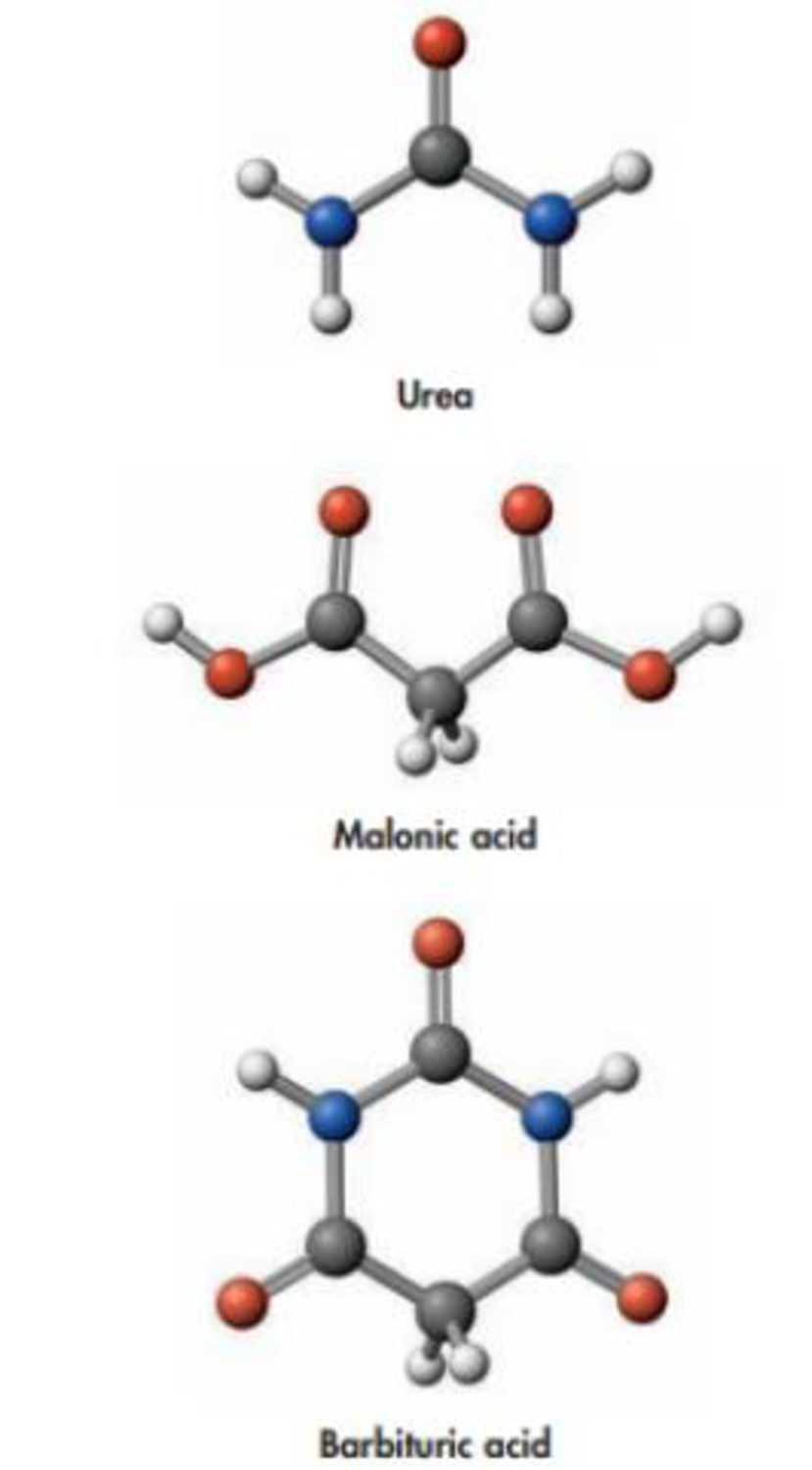Chapter 9, Problem 73SCQ

Chapter
Section
Textbook Problem

Urea reacts with malonic acid to produce barbituric acid, a member of the class of compounds called phenobarbitals, which are widely prescribed as sedatives.(a) What bonds are broken and what bonds are made when malonic acid and urea combine to make barbituric acid? Is the reaction predicted to be exo- or endothermic?(b) Write a balanced equation for the reaction.(c) Specify’ the bond angles in barbituric acid.(d) Give the hybridisation of the C atoms in barbituric acid.(e) What is(are) the most polar bond(s) in barbituric acid?(f) Is the molecule polar?

(a)

Interpretation Introduction

Interpretation:

It should be determined that what bonds are ‘brocken and what bonds are made when malonic acid and urea combine to make barbituric acid. Check whether the reaction is exothermic or endothermic.

Concept Introduction:

Exothermic and endothermic reactions: In this concept exothermic reaction is a chemical reaction that release energy by light or heat. If reaction was absorbed maxima heat energy or light these reaction endothermic reactions.

Explanation

Formation reaction of barbituric acid molecule:

Bond broken reaction:

The two N-H bonds are broken in corresponding urea molecule and two carbonyl (C=O) groups are broken in malonic acid in presence of basic condition to give a cyclic molecule

(b)

Interpretation Introduction

Interpretation:

Balanced equation for the reaction should be written.

Concept Introduction:

• Balanced chemical equation of a reaction is written according to law of conservation of mass.
• Stoichiometry of a chemical reaction is the relation between reactants and products of the reaction and it is represented by the coefficients used for the reactants and products involved in the chemical equation.

(c)

Interpretation Introduction

Interpretation:

Bond angles in barbituric acid should be specified.

Concept Introduction:

Hybridization is the mixing of valence atomic orbitals to get equivalent hybridized orbitals that having similar characteristics and energy.

Geometry of a molecule can be predicted by knowing its hybridization.

TypeofmoleculeHybridaizationGeometryBondangleAX2spLinear180°AX3,AX2Bsp2Trigonalplanar120°AX4,AX3B,AX2B2sp3Tetrahedral109.5°AX5,AX4B,AX3B2,AX2B3sp3dTrigonalbipyramidal120°,90°AX6,AX5B,AX4B2sp3d2Octahedral90°ACentralatomXAtomsbondedtoABNonbondingelectronpairsonA

(d)

Interpretation Introduction

Interpretation:

Hybridization carbon atoms in barbituric acid should be determined.

Concept Introduction:

Hybridization is the mixing of valence atomic orbitals to get equivalent hybridized orbitals that having similar characteristics and energy.

Geometry of a molecule can be predicted by knowing its hybridization.

TypeofmoleculeHybridaizationGeometryBondangleAX2spLinear180°AX3,AX2Bsp2Trigonalplanar120°AX4,AX3B,AX2B2sp3Tetrahedral109.5°AX5,AX4B,AX3B2,AX2B3sp3dTrigonalbipyramidal120°,90°AX6,AX5B,AX4B2sp3d2Octahedral90°ACentralatomXAtomsbondedtoABNonbondingelectronpairsonA

(e)

Interpretation Introduction

Interpretation:

Most polar bond in barbituric acid should be determined.

Concept Introduction:

Polar covalent bond involves an unequal sharing of electrons between two bonded atoms; it creates a partial separation of charge and a dipole moment.

Covalent bonds are polar, if there is a difference in electronegativity between the bonded atoms.

(f)

Interpretation Introduction

Interpretation:

Check whether the barbituric molecule is polar or not.

Concept Introduction:

Polar covalent bond involves an unequal sharing of electrons between two bonded atoms; it creates a partial separation of charge and a dipole moment.

Covalent bonds are polar, if there is a difference in electronegativity between the bonded atoms.

Still sussing out bartleby?

Check out a sample textbook solution.

See a sample solution

The Solution to Your Study Problems

Bartleby provides explanations to thousands of textbook problems written by our experts, many with advanced degrees!

Get Started